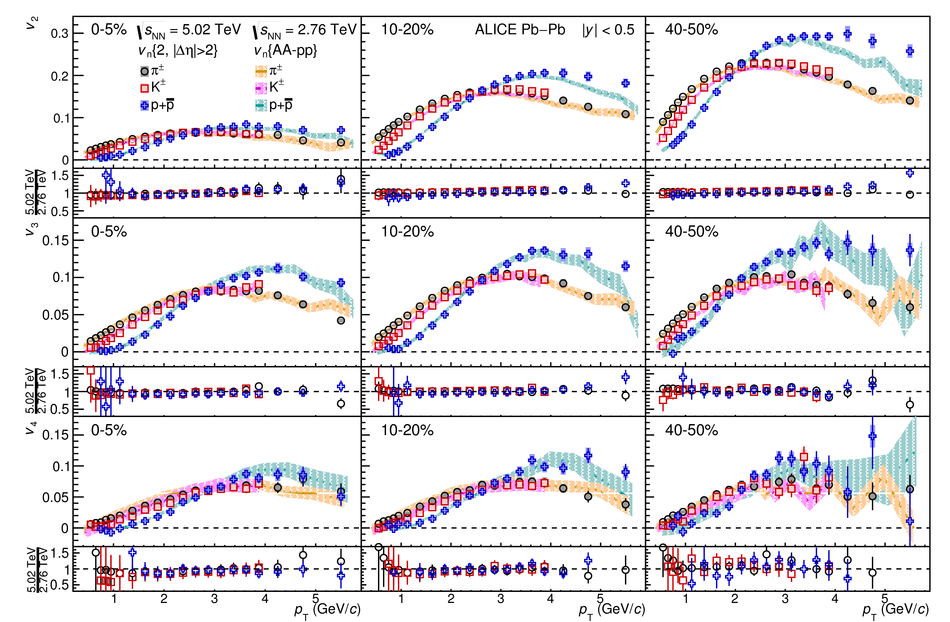# Figure 22

 The $\pt$-differential $v_2$ (top), $v_3$ (middle), and $v_4$ (bottom) of \pipm{}, \kapm{}, and p+\pbar{} compared to ALICE measurements performed in Pb--Pb collisions at \sqrtSnn{} = 2.76~TeV (coloured bands)~ for the 0--5\%, 10--20\%, and 40--50\% centrality classes. For the measurements at \sqrtSnn{} = 5.02~TeV, statistical and systematic uncertainties are shown as bars and boxes, respectively. For the measurements at \sqrtSnn{} = 2.76~TeV, the thickness of the bands corresponds to the quadratic sum of statistical and systematic uncertainties. The ratios of measurements at \sqrtSnn{} = 5.02~TeV to a cubic spline fit to the measurements at \sqrtSnn{} = 2.76~TeV are also presented for clarity.